### Long Division with remainder

#### Example 1      98 ÷ 4 =      can be written as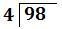First iteration:

 (a) (b) (c) (d) 9 ÷ 4 = 2 4 × 2 = 8 9 − 8 = 1 1 ÷ 4 = number less than one so bring down the number 8 from the original 98: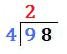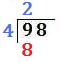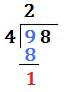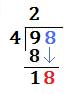Second iteration:

 (a) (b) (c) (d) 18 ÷ 4 = 4 4 × 4 = 16 18 − 16 = 2 2 ÷ 4 = number less than one so bring down the number 0 from 98.0: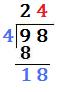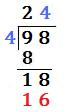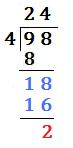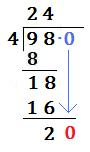Third iteration:

 (a) (b) (c) 20 ÷ 4 = 5 4 × 5 = 20 20 − 20 = 0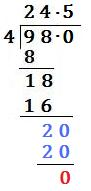So 98 ÷ 4 = 24.5to: